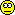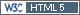ElMaestro
Hero

Denmark,
2018-01-22 23:49

Posting: # 18254
Views: 2,784

## A little off topic: data.frame members [R for BE/BA]

Hi all,there is something about R internals that I don 't understand and I can best exemplify it using some code from power.TOST but my question really isn't about anything in that package.

Imagine I do this in R with powerTOST:

`sampleN.RSABE(CV=0.3, theta0=0.95)`
Nice.
Now, assume I don't want all that intermediate mumbojumbo, and let us for the time being completely forget the print/verbose/details option so I just want the sample size itself as a numeric.

If I inspect the code I see the last few lines:

```    res <- data.frame(design = design, alpha = alpha, CVwT = CVwT,         CVwR = CVwR, theta0 = theta0, theta1 = theta1, theta2 = theta2,         n = n, power = pwr, targetpower = targetpower, nlast = nlast)     names(res) <- c("Design", "alpha", "CVwT", "CVwR", "theta0",         "theta1", "theta2", "Sample size", "Achieved power",         "Target power", "nlast")     if (print | details)         return(invisible(res))     else return(res) ```

...and therefore I can simply do this:
`sampleN.RSABE(CV=0.3, theta0=0.95)\$n`
(the n column is baptised as "Sample size" but is still accessible via \$n)

If I look at the corresponding code snippet from `sampleN.TOST` we have:
```     res <- data.frame(design = design, alpha = alpha, CV = CV,         theta0 = theta0, theta1 = theta1, theta2 = theta2, n = n,         power = pow, targetpower = targetpower)     names(res) <- c("Design", "alpha", "CV", "theta0", "theta1",         "theta2", "Sample size", "Achieved power", "Target power")     if (print)         return(invisible(res))     else return(res) ```
But if I go
`sampleN.TOST(CV=0.3, theta0=0.95)\$n`
then I get NULL.

What determines that difference in behaviour? Why does the res data.frame from both the two functions not hold an extractable \$n ?

Note, this really is solely about R behaviour and R function return/internals (if I want the sample sizes I can also easily get them via [1,7] or [1,8] from the sample size object returned; all this isn't really what this question is about).``` if (3) 4 x=c("Foo", "Bar") b=data.frame(x) typeof(b[,1]) ##aha, integer? b[,1]+1 ##then let me add 1 ```

Best regards,
ElMaestro

"(...) targeted cancer therapies will benefit fewer than 2 percent of the cancer patients they’re aimed at. That reality is often lost on consumers, who are being fed a steady diet of winning anecdotes about miracle cures." New York Times (ed.), June 9, 2018.
Helmut
HeroVienna, Austria,
2018-01-23 13:37

@ ElMaestro
Posting: # 18268
Views: 2,426

## R-Inferno

Hi ElMaestro,

that’s strange. Let’s try to access all values by their (original) names.

```library(PowerTOST) sampleN.RSABE(CV=0.3, theta0=0.95)\$design NULL sampleN.RSABE(CV=0.3, theta0=0.95)\$alpha  0.05 sampleN.RSABE(CV=0.3, theta0=0.95)\$CVwT  0.3 sampleN.RSABE(CV=0.3, theta0=0.95)\$CVwR  0.3 sampleN.RSABE(CV=0.3, theta0=0.95)\$theta0  0.95 sampleN.RSABE(CV=0.3, theta0=0.95)\$theta1  0.8 sampleN.RSABE(CV=0.3, theta0=0.95)\$theta2  1.25 sampleN.RSABE(CV=0.3, theta0=0.95)\$n  27 sampleN.RSABE(CV=0.3, theta0=0.95)\$power NULL sampleN.RSABE(CV=0.3, theta0=0.95)\$targetpower NULL sampleN.RSABE(CV=0.3, theta0=0.95)\$nlast  27 sampleN.TOST(CV=0.3, theta0=0.95)\$design NULL sampleN.TOST(CV=0.3, theta0=0.95)\$alpha  0.05 sampleN.TOST(CV=0.3, theta0=0.95)\$CV  0.3 sampleN.TOST(CV=0.3, theta0=0.95)\$theta0  0.95 sampleN.TOST(CV=0.3, theta0=0.95)\$theta1  0.8 sampleN.TOST(CV=0.3, theta0=0.95)\$theta2  1.25 sampleN.TOST(CV=0.3, theta0=0.95)\$n NULL sampleN.TOST(CV=0.3, theta0=0.95)\$power NULL sampleN.TOST(CV=0.3, theta0=0.95)\$targetpower NULL```

We get some NULLs also from `sample.RSABE()`. Even more interesting calling a variable which does not exist (since R is case-sensitive). `foo()\$design` gave NULL, but:

```sampleN.RSABE(CV=0.3, theta0=0.95)\$Design  2x3x3 Levels: 2x3x3 sampleN.TOST(CV=0.3, theta0=0.95)\$Design  2x2 Levels: 2x2```

In the functions of `PowerTOST` `design` is character variable, but `Design` is a 1-level factor:
```is.factor(sampleN.TOST(CV=0.3, theta0=0.95)\$Design)  TRUE```

I don’t get it.

» […] if I want the sample sizes I can also easily get them via [1,7] or [1,8] from the sample size object returned …

I suggest to use `Sample.Nfoo()[["Sample size"]]` instead. You can use it in all functions and the current indices are not carved in stone. If we introduce another variable before #7 or reorder variables in a future version of `PowerTOST` your code will not work any more.

Cheers,
Helmut SchützThe quality of responses received is directly proportional to the quality of the question asked. ☼
Science Quotes
d_labes
Hero

Berlin, Germany,
2018-01-23 15:03

@ Helmut
Posting: # 18270
Views: 2,319

## R-Inferno

Hi Helmut, hi ElMaestro,

» that’s strange...
In deed!

The answer to that problem:
R is an interactive language and tries to save the user from keystrokes.
Partial match of names is one of these tries.
See Patrick Burns R-Inferno, Paragraph "8.1.20 partial matching can partially confuse".

That means what we see in case of `power.RSABE()\$n` is the value of `power.RSABE()\$nlast`.
Try:
```r2 <- sampleN.RSABE(CV=0.3, theta0=0.95, print=F) r2\$n # will give 27, the original nlast r2\$nlast <- 100 r2\$n # will give our changed content nlast=100```

`sampleN.TOST()` doesn't have a return parameter which could be matched with n.
Thefore we obtain correctly NULL.

» ... In the functions of `PowerTOST` `design` is character variable, but `Design` is a 1-level factor.
Thats the known crazy default behaviour if character variables are incorporated in data.frames.
The lazy author of the functions had forgotten to use the argument `stringsAsFactors` in creating the return data.frame. Have his butt's. Also for capitalizing Design.

Regards,

Detlew
ElMaestro
Hero

Denmark,
2018-01-23 15:26

@ d_labes
Posting: # 18272
Views: 2,377

## Thanks :-D

What a fantastic little story. Mysteri solved. Thank you, Hötzi, for confirming that I am not completely hopeless. And thank you, d_labes, for confirming that I partially amConclusion: from this point onwards I will do e.g. `sampleN.RSABE(CV=0.3, theta0=0.95)\$Sa` etc.

This code apparently illustrates the phenomenon:

A=c("foo", "bar")
B=c(1:2)
C=data.frame(A,B)
names(C)=c("Banana", "Boxer")
C\$B
C\$Bo
C\$Ba

Now I can have peace of mind. Or closure, no pun intended.

``` if (3) 4 x=c("Foo", "Bar") b=data.frame(x) typeof(b[,1]) ##aha, integer? b[,1]+1 ##then let me add 1 ```

Best regards,
ElMaestro

"(...) targeted cancer therapies will benefit fewer than 2 percent of the cancer patients they’re aimed at. That reality is often lost on consumers, who are being fed a steady diet of winning anecdotes about miracle cures." New York Times (ed.), June 9, 2018.
d_labes
Hero

Berlin, Germany,
2018-01-23 15:39

@ ElMaestro
Posting: # 18273
Views: 2,325

## Safe side syntax

Dear ElMaestro,

» Conclusion: from this point onwards I will do e.g. `sampleN.RSABE(CV=0.3, theta0=0.95)\$Sa` etc.

to be on the safe side use `sampleN.RSABE(CV=0.3, theta0=0.95, print=F)\$`Sample size``.
Ugly. But you get always what you want. Contrary to the Stones.

Regards,

Detlew
yjlee168
SeniorKaohsiung, Taiwan,
2018-01-23 17:47

@ ElMaestro
Posting: # 18279
Views: 2,378

## A little off topic: data.frame members

Hi all,

I think we can use str() to preview all factors' name in a data.frame.

```df <- sampleN.TOST(CV=0.3, theta0=0.95) df\$n NULL str(df) 'data.frame':   1 obs. of  9 variables:  \$ Design        : Factor w/ 1 level "2x2": 1  \$ alpha         : num 0.05  \$ CV            : num 0.3  \$ theta0        : num 0.95  \$ theta1        : num 0.8  \$ theta2        : num 1.25  \$ Sample size   : num 40  \$ Achieved power: num 0.816  \$ Target power  : num 0.8 ```
There is no 'n' in df or sampleN.TOST(CV=0.3, theta0=0.95). So it returns NULL. If we do

```df['Sample size']   Sample size 1          40 df['Target power']   Target power 1          0.8 sampleN.TOST(CV=0.3, theta0=0.95)['Sample size']  +++++++++++ Equivalence test - TOST +++++++++++             Sample size estimation ----------------------------------------------- Study design:  2x2 crossover log-transformed data (multiplicative model) alpha = 0.05, target power = 0.8 BE margins = 0.8 ... 1.25 True ratio = 0.95,  CV = 0.3 Sample size (total)  n     power 40   0.815845   Sample size 1          40 ```
then it works.

» ...and therefore I can simply do this:
» `sampleN.RSABE(CV=0.3, theta0=0.95)\$n`
» (the n column is baptised as "Sample size" but is still accessible via \$n)

All the best,
---Yung-jin Lee
bear v2.8.3-3:- created by Hsin-ya Lee & Yung-jin Lee
Kaohsiung, Taiwan http://pkpd.kmu.edu.tw/bearIng. Helmut Schütz